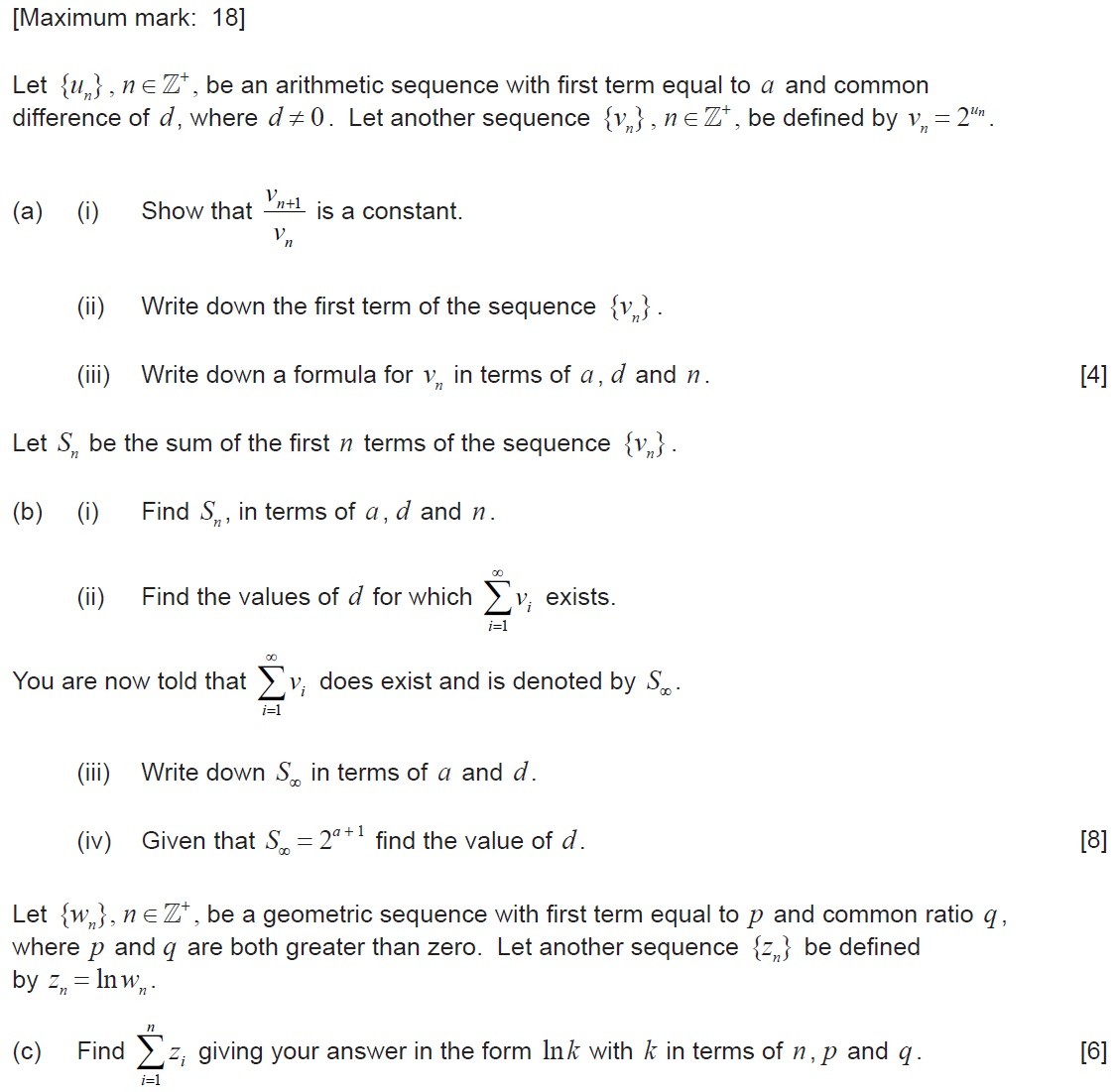# IBDP Past Year Exam Questions – Sequences and Series

1. [M04/P1]

A geometric series has a negative common ratio. The sum of the first two terms is 6. The sum to infinity is 8. Find the common ratio and the first term.

2. [M04/P2]

The three terms a, 1, b are in arithmetic progression. The three terms 1, a, b are in geometric progression. Find the value of a and of b given that a ≠ b .

3. [M99/P1]

The second term of an arithmetic sequence is 7. The sum of the first four terms of the arithmetic sequence is 12. Find the first term, $a$ , and the common difference,  $d$ , of the sequence.

4. [M99/P2]

The ratio of the fifth term to the twelfth term of a sequence in an arithmetic progression is  $\frac{6}{13}$ . If each term of this sequence is positive, and the product of the first term and the third term is 32, find the sum of the first 100 terms of this sequence.   [7 marks]

5. [M98/P1]

The first, second and the  $n$ the terms of an arithmetic sequence are 2 , 6 , and 58 , respectively.

(a) Find the value of  $n$ .

(b) For that value of  $n$ , find the exact value of the sum of  $n$ terms of a geometric sequence whose first term is 2 and common ratio is  $\frac{1}{2}$ .

6. [M08/P1/TZ1]

A circular disc is cut into twelve sectors whose areas are in an arithmetic sequence. The angle of the largest sector is twice the angle of the smallest sector.
Find the size of the angle of the smallest sector.

7. [N08/P1/TZ0]

An 81 metre rope is cut into n pieces of increasing lengths that form an arithmetic sequence with a common difference of d metres. Given that the lengths of the shortest and longest pieces are 1.5 metres and 7.5 metres respectively, find the values of n and d .

8. [N08/P2/TZ0]

A geometric sequence has a first term of 2 and a common ratio of 1.05. Find the value of the smallest term which is greater than 500.

9. [N12/P2/TZ0]

Find the sum of all the multiples of 3 between 100 and 500.   [4 marks]

10. [N15/P1/TZ1]11. [M95/P2]

(a) The fifth, seventh and twelfth terms of the arithmetic sequence  are consecutive terms of a geometric sequence. Find the common ratio of the geometric sequence.   [9 marks]

(b) The sum of first  $k$ positive integers can be written as

$1+2+3+.......+k=\frac{k\left(k+1\right)}{2}$ .

Given  $n\in \mathrm{ℕ}$ find, in terms of  $n$ , the sum of the integers between 1 and  $15n$ inclusive which are not divisible by 3 or 5. Simplify your answer as much as possible.   [9 marks]